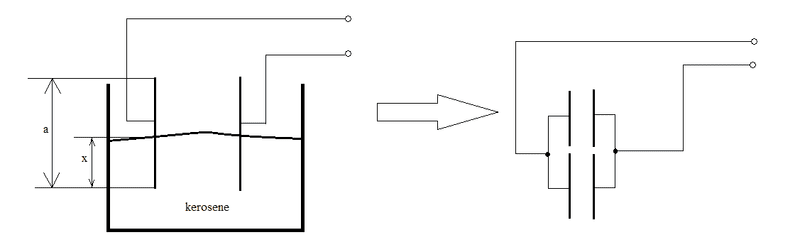# The value of electric current on a sinking conductor

• darkprior

## Homework Statement

A flat capacitor formed by two square plates of side 0.3 m which are 2 mm apart. Source keeps voltage 250 V on the plates. What current flows between the plates and the source if the condenser is immersing in kerosene at velocity of 5 mm / s? The relative permittivity of kerosene is 2.

-

## The Attempt at a Solution

Here is a picI=Q/t

Because of the fact that immersing cunductor can be understood as two parallel conductors the capacity of an immersed conductor (the depth x = v*t) is C =(ε0*(a-x)*a)/d + (ε0εr*a*x)/d

My question is how do I compute the time in the x = v*t? And sorry for my english.

The simple answer is you don't. Just assume you know it and hope it cancels out in the end.

The simple answer is you don't. Just assume you know it and hope it cancels out in the end.
But it doesn't cancel in the end because of the plus and minus in the equation

What equation? Remember your ultimate goal is to find the current.

What equation? Remember your ultimate goal is to find the current.
I think I get it now...

I put the "v*t" instead the "x" in the equation for capacity, multiply it by the voltage (U) and then derivate it following I=dQ/dt

Or am I wrong?

Anyways thank you for your clear explanation, it helped me a lot

Last edited:
That's right. Or you could just leave it as ##x##, and when you differentiate with respect to time, you'll get ##v## in its place.